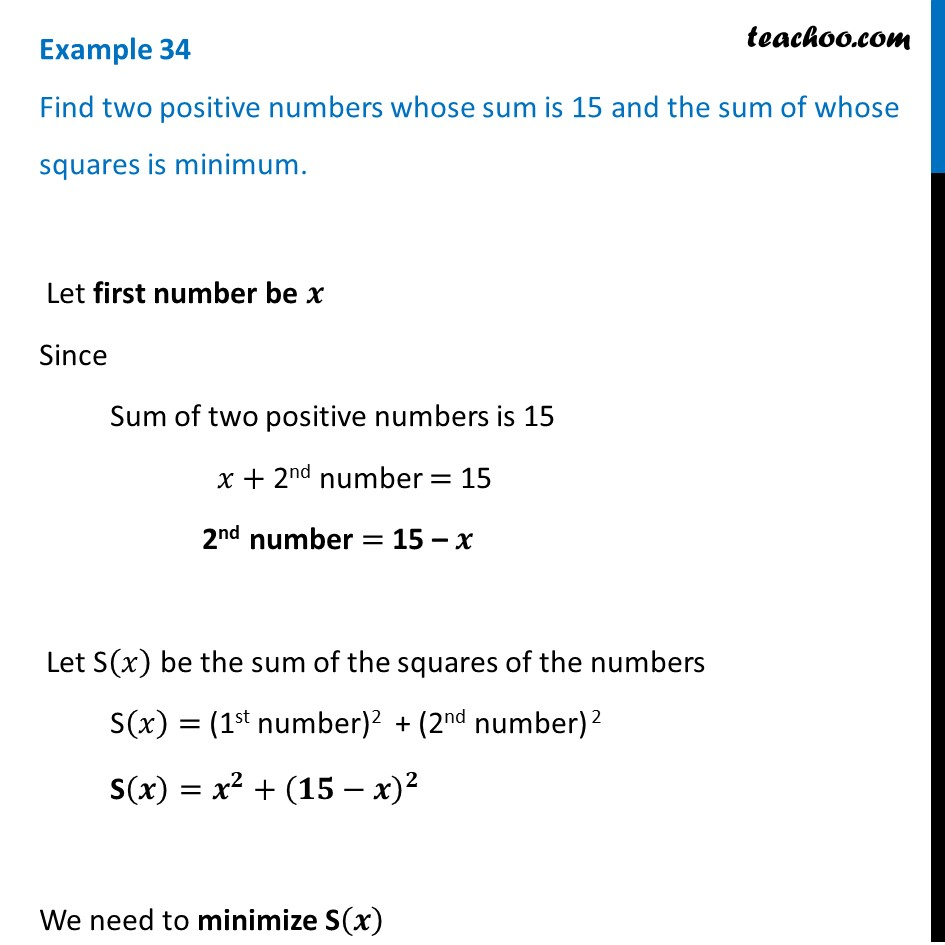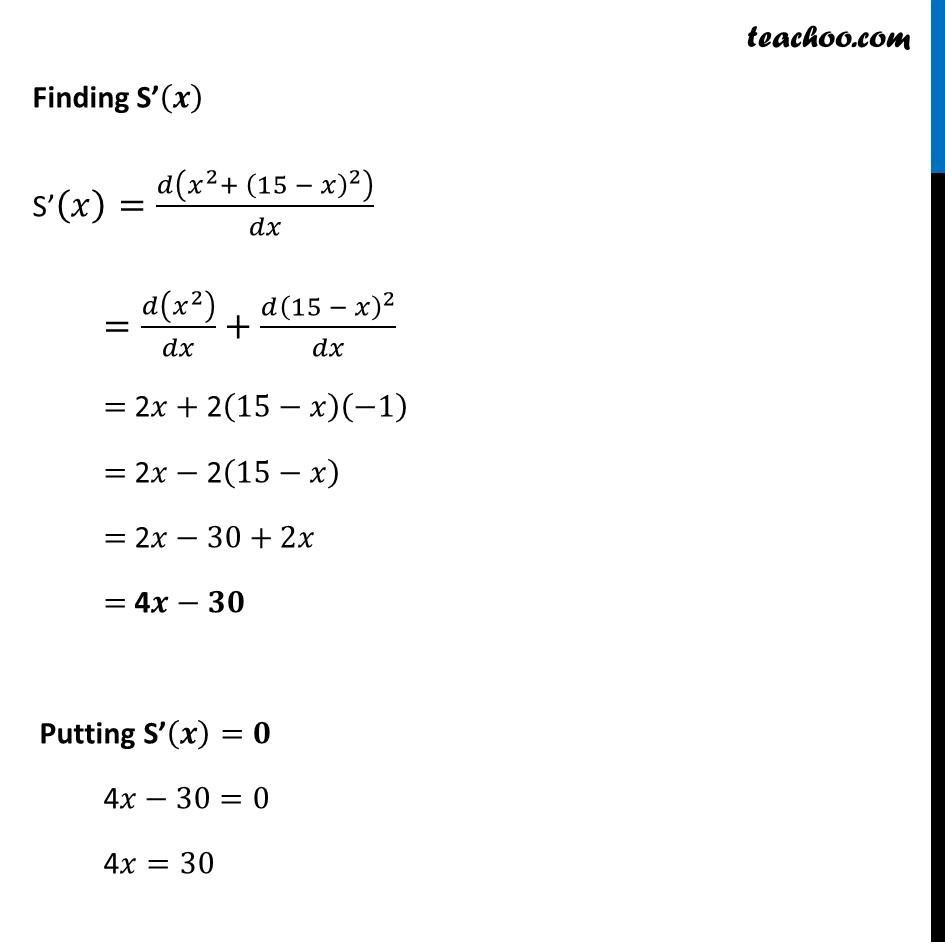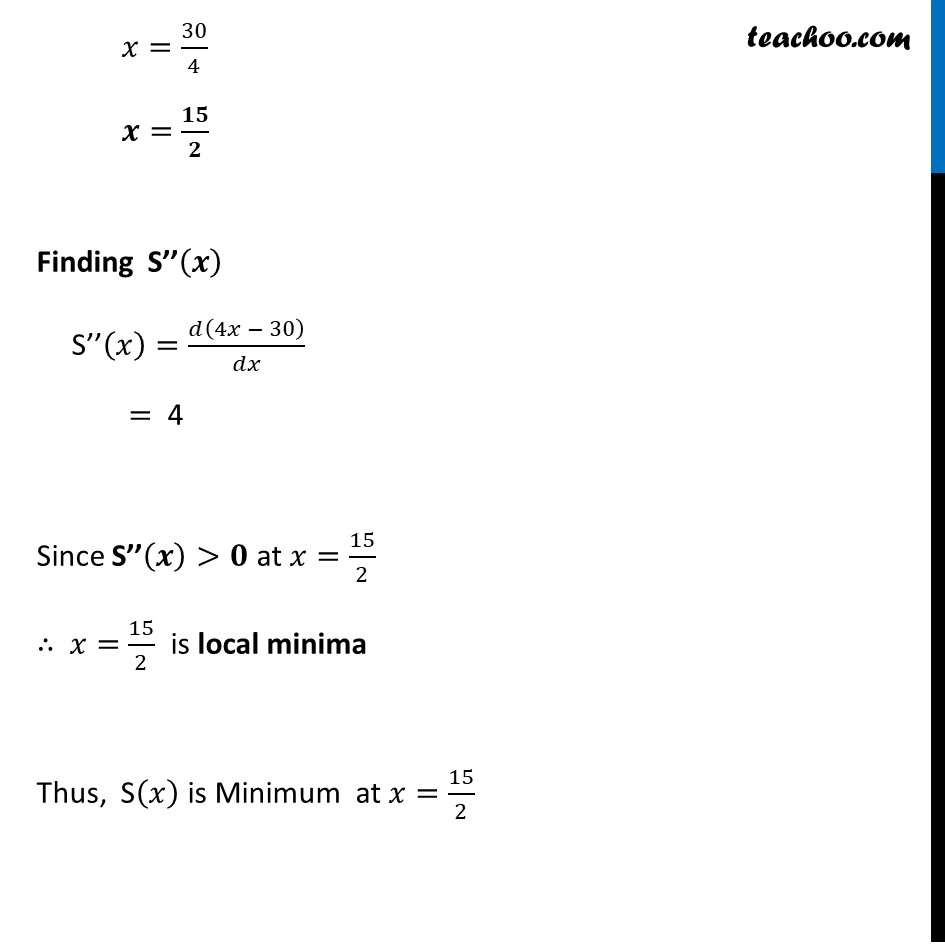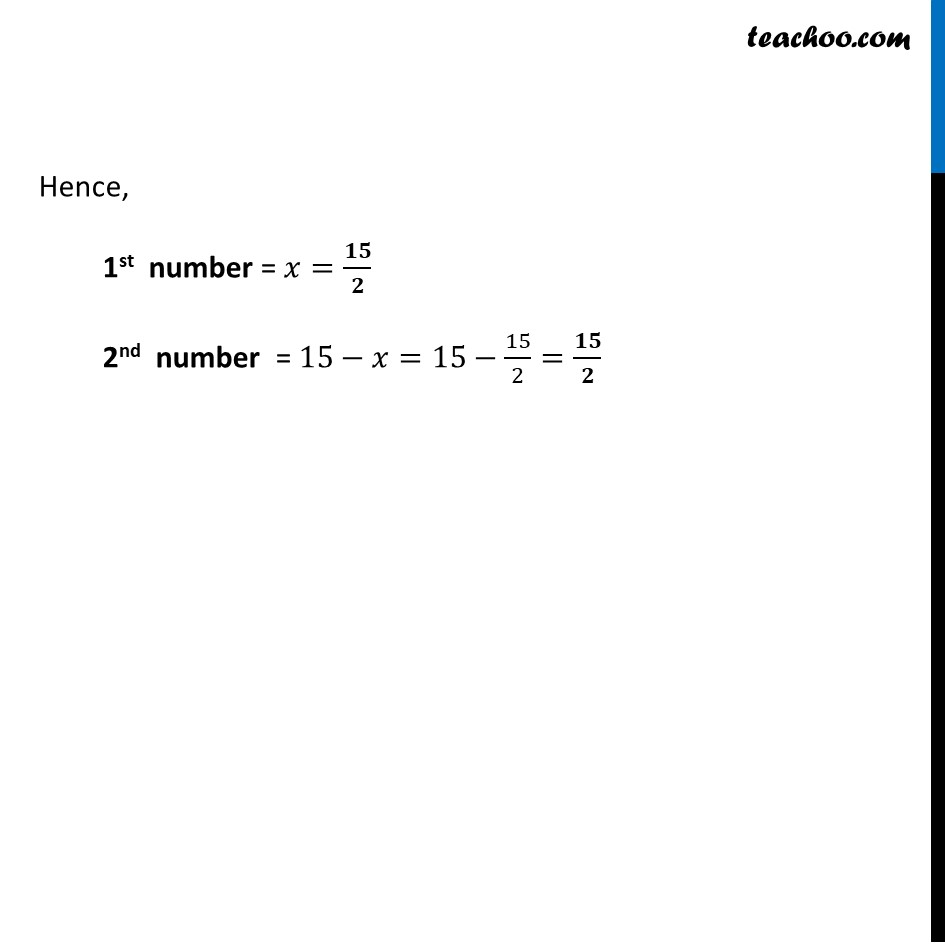Examples

Chapter 6 Class 12 Application of Derivatives
Serial order wiseLearn in your speed, with individual attention - Teachoo Maths 1-on-1 Class

### Transcript

Example 22 Find two positive numbers whose sum is 15 and the sum of whose squares is minimum. Let first number be 𝒙 Since Sum of two positive numbers is 15 𝑥+ 2nd number = 15 2nd number = 15 – 𝒙 Let S(𝑥) be the sum of the squares of the numbers S(𝑥)= (1st number)2 + (2nd number) 2 S(𝒙)=𝒙^𝟐+(𝟏𝟓−𝒙)^𝟐 We need to minimize S(𝒙) Finding S’(𝒙) S’(𝑥)=𝑑(𝑥^2+ (15 − 𝑥)^2 )/𝑑𝑥 =𝑑(𝑥^2 )/𝑑𝑥+(𝑑(15 − 𝑥)^2)/𝑑𝑥 = 2𝑥+ 2(15−𝑥)(−1) = 2𝑥− 2(15−𝑥) = 2𝑥−30+2𝑥 = 4𝒙−𝟑𝟎 Putting S’(𝒙)=𝟎 4𝑥−30=0 4𝑥=30 𝑥=30/4 𝒙=𝟏𝟓/𝟐 Finding S’’(𝒙) S’’(𝑥)=𝑑(4𝑥 − 30)/𝑑𝑥 = 4 Since S’’(𝒙)>𝟎 at 𝑥=15/2 ∴ 𝑥=15/2 is local minima Thus, S(𝑥) is Minimum at 𝑥=15/2 Hence, 1st number = 𝑥=𝟏𝟓/𝟐 2nd number = 15−𝑥=15−15/2=𝟏𝟓/𝟐